# Team:KAIST Korea/Project Modeling

KAIST Korea 2012 iGEM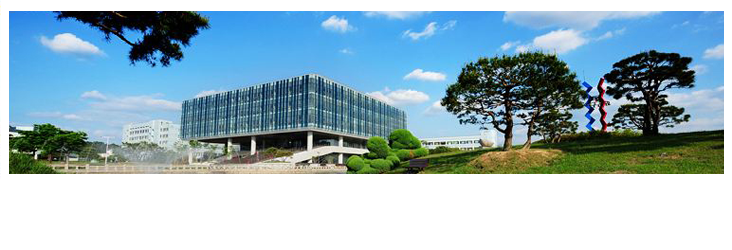Project : ModelingCell Growth Curve Proof of concept Auto Regulation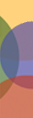# Modeling

Computational modeling of our project

Cell Growth Curve

Cell growth can be modeled using Logistic differential equation as shown below.When we solve this equation with appropriate parameters(using MATLAB), we can get solution curve as shown below. This curve matches with our knowledge about cell growth.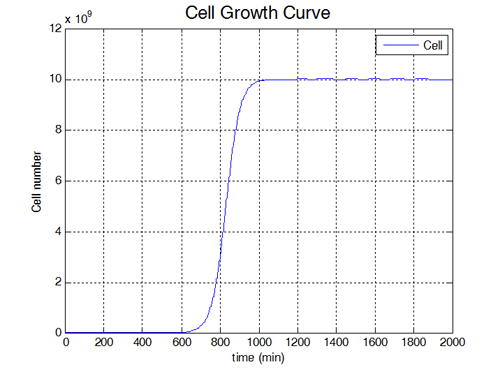Proof of concept

• Mathematical model

With GFP and RFP, we want to check whether our system really works or not. Since we cannot consider all the complicated biological phenomena, we assume our system simply follows reaction rate theory and mass balance equations. Reactions below are simplified version of our system. We CONSIDERED the production and degradation of mRNAs and Genes, while we IGNORED the polysome phenomena and any gene regulatory system that occurs in real biological system. Also, we CONSIDERED that there are many copies of plasmids in E.coli and each plasmid can react with invertase to invert their gene sequence.
Because every plasmid has equal probability to react with invertase, we assume plasmids follow uniform distribution. Final assumption is that each plasmid is mutually independent, that is, each plasmid cannot affect invertase reaction of other plasmid.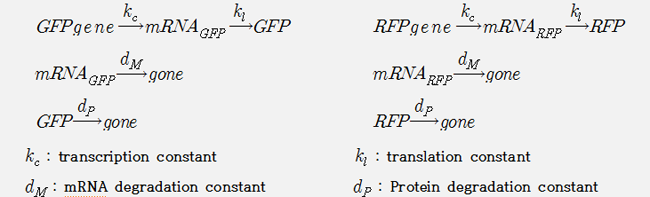Using these reaction, we constructed mathematical model of our system as shown below. Pg(gene probability), in our model, represents the number of plasmid which is inverted. And the rate of producing inverted gene is reduced as the remaining non-inverted gene is reduced.Using MATLAB we can solve these set of differential equations and get solution curve like below.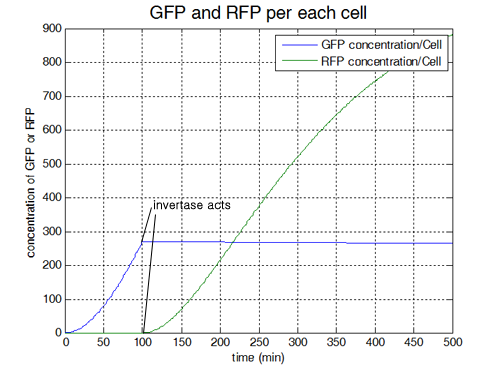We also consider concentration of GFP/RFP of cell colony. The solution curve shown below represents that result.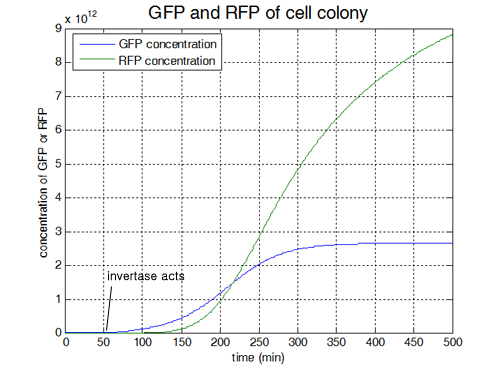• Parameter Sensitivity Analysis

We also did parameter sensitivity analysis to find what kind of parameters critically impact on our system. We defined sensitivity coefficient and calculated as paramters vary with some ratio.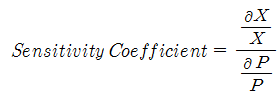And we plot the result using 3D bar graph. As graph represents, some parameters are critical to change the output of our system and some are not.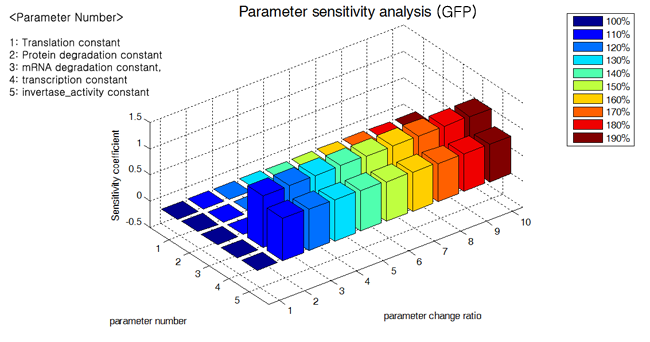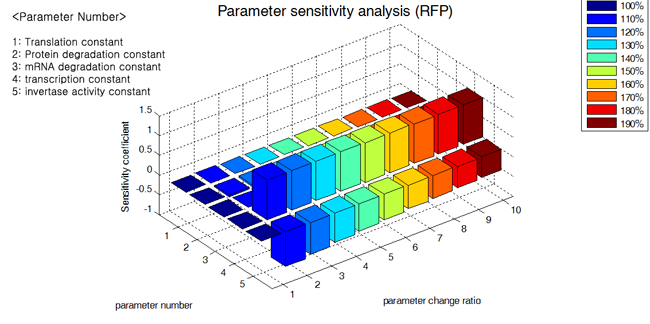Auto Regulation

• Mathematical model

Similar to 'Proof of concept, mathematical model', we can write simplified equation of our system, that is shown below.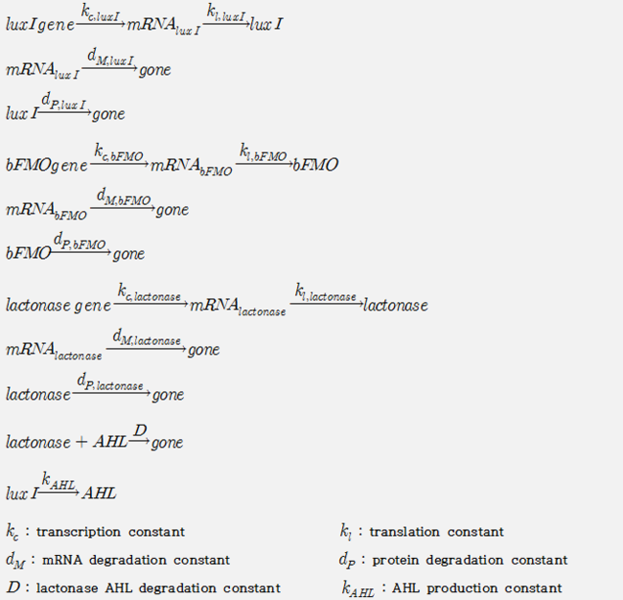Based on the reaction above, we constructed our mathematical model like this.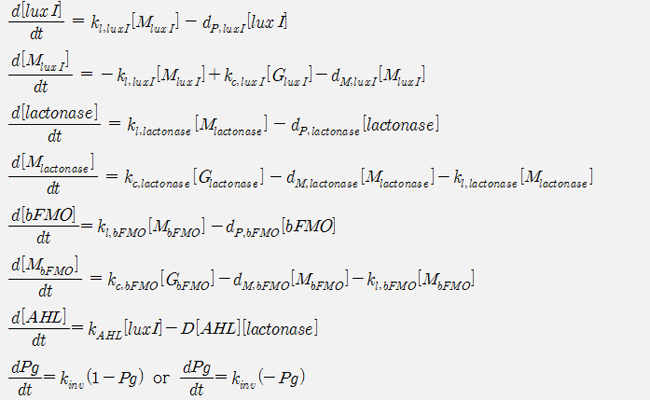Using MATLAB we can solve these set of differential equations and get solution curve like below.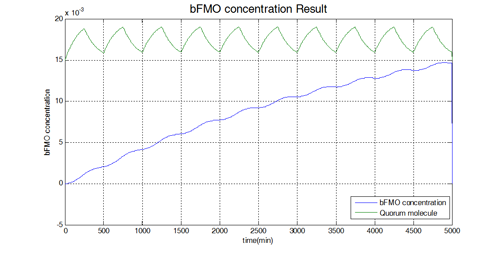When we consider the cell colony instead of one cell, we simply multiply cell growth curve to original result. And derived curve is like below.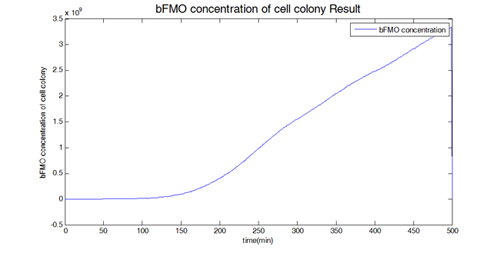• Parameter Sensitivity Analysis

We also did parameter sensitivity analysis and the result is shown below.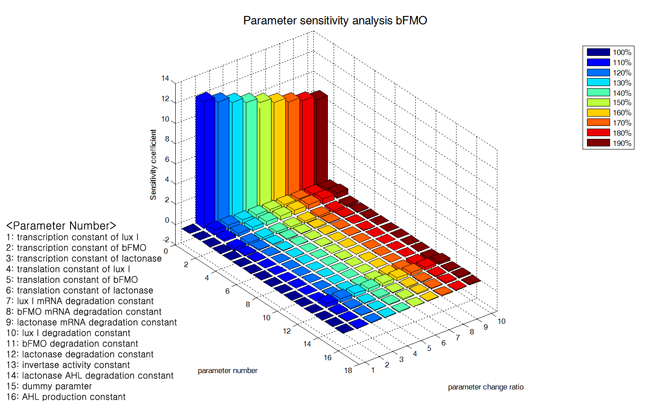Kaist Footer